# Velocity Perturbation

The flux-freezing constraint of marginally-stable ideal-MHD takes the form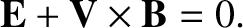(3.55)

[See Equation (2.379).] Taking the curl of the previous equation, combining with Maxwell's equations, and linearizing, we obtain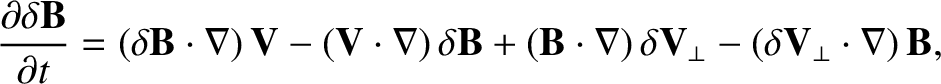(3.56)

where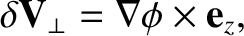(3.57)

and use has been made of Equation (3.50), as well as the facts that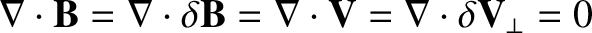. The radial component of the previous equation yields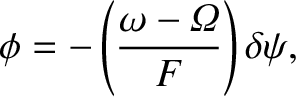(3.58)

where use has been made of Equations (3.9), (3.19), (3.32), (3.52), and (3.53). Equations (3.54) and (3.58) imply that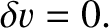(3.59)

Hence, we deduce that the perturbed plasma flow associated with a tearing mode in a low-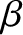, large aspect-ratio, tokamak plasma is divergence-free [see Equations (3.51) and (3.59)], and is specified by Equations (3.57) and (3.58).